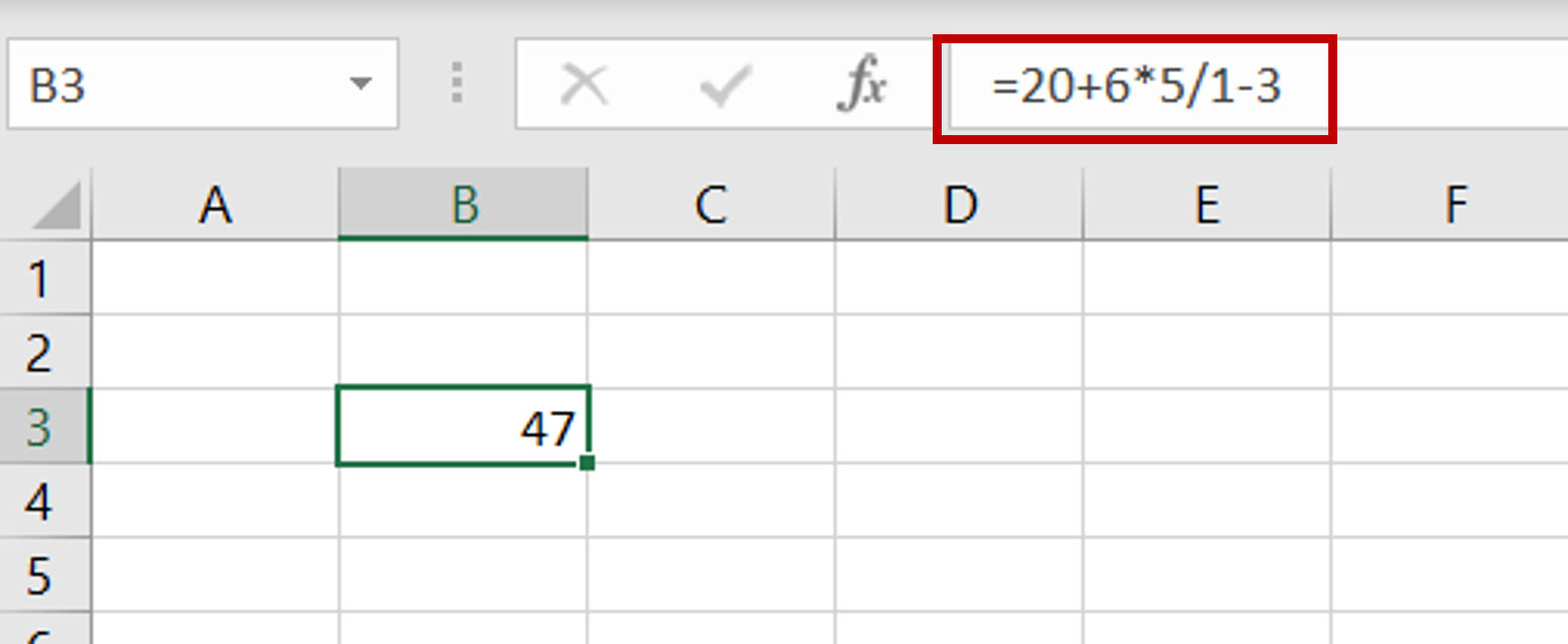# How to use Excel as a calculator

You can watch a video tutorial here.Excel can be used for all the functions that we use a calculator for.  When you use Excel as a calculator, the mathematical operation is entered in a cell as a formula and this can then be copied to other cells. Basic operations such as add, subtract, multiply and divide can be used and these can be extended to more complex formulas using functions. Using an equal sign (=) tells Excel to treat the numbers and symbols as mathematical operators.

### Step 1 – Type the numbers preceded by ‘=’– Select a cell
– Type ‘=’ followed by the calculation to be done
– Press Enter
Note: Excel largely follows the BODMAS rules to determine the order in which the numbers are executed so you will need to use brackets when there are multiple operators.